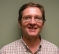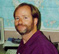## simple and accurate phase estimation of harmonics in a signal?Started by 6 years ago16 replieslatest reply 6 years ago799 views

I'm trying to model each of the harmonics of a fundamental carrier as a sine wave with an accurate amplitude and phase.

I know certain windows can be used to extract the amplitude accurately. But what about phase?

Are there any simple techniques to extract the phase of a harmonic more accurately than just taking an FFT and computing atan(imag/real)?

[ - ]I suppose the question is how accurately do you know or can you measure the frequency of the fundamental carrier?  If you know that then cross-correlation (windowed) of generated sin/cos waves can probably give you a better answer.  It is like a discrete DFT at specific frequencies.  The frequencies do not have to be on exact multiples like a true DFT. There are several ways to do this.  Some fairly clever.  The sliding DFT architecture comes to mind.  Brute force approach uses long correlation tables.

Its been over 30 years ago now that we measured received waveforms this way in an airborne geophysical instrument design.  Processing was done by the 80286 CPU pushing A/D data at 8 MHz (remember the "out string" instruction?) onto several parallel cards each with sin/cos LSI multiply-accumulators and SRAM lookup tables holding the cross coefficients.  Crazy to even think about now.  We could get the in-phase/quadrature measurements accurate to within 1 PPM.  However, the system ran synchronously and we used synchronous detection so the frequency match was exact.

If you don't have the ability to lock your sample rate to some known frequency relationship of your fundamental then can you measure it very accurately?  There is a paper from IEEE DSP Tip and Tricks which covers frequency estimation from an FFT.  The harmonic tables could be derived from that measurement.

Another way for an ongoing measurement is to lock a digital PLL to the fundamental frequency (might have to tightly filter it, then Hilbert transform).  This can be very, very accurate.  Then you can use the PLL's NCO (or a Fractionally Derived NCO, another good subject) to drive a Farrow filter (assuming that your total signal band-width is limited).  I've used a Farrow filter design that was good out to almost Fs/4.  Regardless, you need a Farrow filter that is appropriate for your bandwidth.  The metrics from the PLL will tell you the accuracy and stability of the fundamental measurement.  The output from the Farrow filter will have the harmonics on exact frequencies so again sliding DFTs or long windowed cross correlations will give the mag/phase measurements.  Really depends on how frequency stable your signal is.

Also, the time length of the window (integration interval) and noise level of the system govern the accuracy of the measurements.

Probably more than you wanted to know.

Best regards,

Mark Napier

[ - ]Or this if your signal is clean enough:

Looks like you will have to filter each of the harmonics to get a very clean tone and measure each one separately.

[ - ]Thanks for the reference to my article, but it is not appropriate for this situation.  Even if you do separate out all the tones, my article is about finding the frequency whereas the OP needs to find the phase.

The DFT is a much better approach in this situation.

Ced

[ - ]Keeping things simple, making a few assumptions, and building on your idea of using the FFT--

A problem you will have with the FFT is that the frequency of your sine waves will fall between the frequency spectrum samples. You can greatly improve this by padding the time domain segment with zeros before taking the FFT.  For instance, take 1024 points from the signal, add zeros to the end to make it 1024 x 64 = 65536, take a N=65536 FFT, find the locations of the peaks, find the corresponding phases, and calculate the phase shift between the fundamental and harmonics. You then might want to repeat the process multiple times, averaging all of the measured phase shifts to reduce noise.   You can think of the zero padding as interpolating between the frequency spectrum data points to give you a better estimate of where the peak actually is.

Alternatively, you can apply a flat-top window before taking the FFT.  This makes the peak in the frequency spectrum have a flat-top of several data points, preventing anything from falling between the cracks. It is mainly used to get a more accurate amplitude measurement.   I've never done it, but I suspect that this allows the corresponding phase to be measured more precisely as well, since the phase will be approximately a constant over these several data points. Here's a reference to the flat-top window: http://www.dspguide.com/CH9.PDF (see Fig. 9-5).

[ - ]Thanks Mark and Steve, My sample size is quite large (can go up to 1e9 pts). I'm currently using a flat-top window for accurate amplitude and it works great. I'm not doing anything special for phase, so I thought that might be the largest source of error. I have the waveforms up on another posting here,

I thought I could extract a model from the FFT data as shown in that posting, but the fit is still poor. The advantage of that approach is the shape and period are the same in EVERY cycle, which is what I require. Although, I'm not exactly confident that I've mapped the FFT data into my 'model' correctly.

[ - ]Your situation is the kind of application for which the DFT is ideal.  What you want to do is select a frame size that is a whole multiple of your repeating pattern.  Let's assume five.  Next you will want to select your sampling density so there are about four samples per every cycle of the highest harmonic you choose.  You said you went up to 20 so there will be 100 (5 X 20) cycles of your highest harmonic in the frame.  Therefore you will want about 400 samples.  You can round that up to 512 if you want to do a FFT.

Having a whole number of waveforms in your frame makes the pertinent information fall in an evenly spaced set of bins.  For the case of five, the bins of interest will be 0, 5, 10, 15, ..., 100.  Instead of doing a full DFT or FFT, you only need to calculate these bins as they are the only ones you will use for your series.

Secondly, what I suspect may be the cause of your inaccuracy, is that you will want to use the atan2(imag,real) function rather than atan(imag/real).

To get a better fit, use more harmonics.  With a whole number of waveforms in your frame, the Fourier series you derive and the inverse DFT are equivalent.

Hope this helps,

Ced

[ - ]Thanks Cedron, There is some noise in the waveform. I can try to get, say, 100 "whole" cycles, but the starting and ending points may not perfectly be aligned in their respective cycles (due to noise). Wouldn't that require windowing, or will it just introduce lower-frequency noise in the spectrum (on order of 1/100 cycles) and thus won't interfere with the harmonic analysis?

[ - ]You're welcome.

I think 100 waveforms may be overkill and you will need to do a huge FFT.  The simplest (at least conceptually) way to find a frequency in the time domain is to look at zero crossings (of the same type relative to the waveform).

Your first step is to get an accurate assessment of your base frequency so you can select the appropriate frame size.  So using 100 waveforms to find how many samples per waveform you have should give you an accurate enough answer to use on fewer waveforms.

You can mitigate the effect of the noise by applying the technique I describe in my article "Exponential Smoothing with a Wrinkle":

https://www.dsprelated.com/showarticle/896.php

You can use the smoothed signal to better find the waveform size in samples.  You can then do the DFT on the smoothed signal (choose your frame to avoid the ends of your smoothing range) to find the phase values.  The article also provides formulas for converting the smoothed amplitudes to the raw amplitudes.

I wouldn't bother with that though, based on what your graph looks like in the other posting.  If you get the base frequency correct within a sample or two on 100 waveforms the results should be accurate enough.

Five waveforms is a good number.  You want at least two and I don't see a whole lot of benefit by going over ten at the cost of much larger DFTs.  (Unless you are only calculating only the bins you need, then the more the merrier assuming your waveforms stay consistent.)  Any values in the other bins will be due to noise.

To clarify a little bit on my other answer.  You want at least four points for your highest harmonic (as opposed to the Nyquist value of two) but a higher sampling density won't hurt.

You do not want to use any window functions, you are interested in the Fourier coefficients.

Ced

[ - ]That helps, I see what you are trying to do.  I'm nearly certain that your problem is a bug in the code, not an accuracy issue in determining the phase. You are using a canned FFT routine to move from the time domain to the frequency domain. This is an implementation of the complex DFT.  Afterward, you use your own code for the inverse transform to get from the frequency domain back to the time domain.  Your code is based on the real DFT.

The problem is that the complex and real DFTs have some small differences in how they are defined.  These are only a couple of sign and scaling issues, but they cause chaos if not accounted for, and they can drive you crazy finding them.

My suggestion is to start with something like a computer generated sawtooth wave.  Take the FFT, and then try to recreate the sawtooth by using all of the frequency domain coefficients with your homebrew inverse DFT. My guess is that it is some kind of sign error on the phase. Here's a couple of links on moving between the real and complex DFTs.

[ - ]or better yet, use a computer generated sine wave instead of a sawtooth.

[ - ]I disagree.  Judging by the second diagram in his StackExchange posting, it seems to me that he has the fundamental frequency and phase stuff pretty well figured out correctly.  I think his error in his "home brewed" inverse DFT, aka "Finding Fourier coefficients from DFT bin values", lies in the use of atan(imag/real) instead of the atan2(imag,real) as I stated before.  What has probably happened is that some of his phase values are 180 degrees (Pi radians, one half wavelength) out of phase.

If atan2 is unavailable he needs to employ some if logic as shown here:

Ced

[ - ]Could be... that's a common bug that produces a sign error on the phase.

[ - ]Did you figure it out?

Ced

[ - ]Yes, it was the atan() versus atan2() phase errors. Thanks so much for pointing it out Cedron.

[ - ]